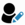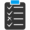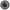Core Java - Interview Questions and Answers for 'If statement' | Search Java Interview Question - javasearch.buggybread.com# Search Interview Questions3356 questions in repository.There are more than 200 unanswered questions.Have a video suggestion.
Click Correct / Improve and please let us know.
Label / Company      Label / Company / TextSubmit Question

Core Java - Interview Questions and Answers for 'If statement' - 3 question(s) found - Order By NewestQ1. What is wrong with the following if statement ?

if(x==y && x.equals(y) {
}
Core Java2016-07-28 13:45:21Ans. x==y means that both references have same type and are pointing to same memory location and hence would always mean that they have same value.

x.equals(y) is not required in this case.Help us improve. Please let us know the company, where you were asked this question :LikeDiscussCorrect / Improveif statement  control statements  == and equals  ==  .equals   code optimizationTry 2 Question(s) TestRelated QuestionsDifference between == and .equals() ?What is the difference between = and == in Java ?How is == operator different for objects and primitive types ?What will be the output of following code

Integer x = 1;
Integer y = 2;
System.out.println(x == y);

What if you change 1 to "1" and Integer to String?Q2. What is wrong with the following if statement ?

if(x==y || x.equals(y) {
}
Core Java2016-07-28 13:48:23Ans. if x==y turns out to be true x.equals(y) will be true too. If x.equals(y) could be true even if x==y is true or not.

So the only possible outcomes are

1 || 1 = 1
0 || 1 = 1
0 || 0 = 0

i.e the outcome of x.equals(y)

check for x==y is not required in this if statement.Help us improve. Please let us know the company, where you were asked this question :LikeDiscussCorrect / Improveif statement  control statements  == and equals  ==  .equals   code optimizationTry 1 Question(s) TestRelated QuestionsDifference between == and .equals() ?What is the difference between = and == in Java ?How is == operator different for objects and primitive types ?What will be the output of following code

Integer x = 1;
Integer y = 2;
System.out.println(x == y);

What if you change 1 to "1" and Integer to String?Q3. What is nested if ?Core Java2017-07-24 13:11:45Ans. if statements nested within another if or else blocks are called nested if statements.

if(condition1) {
if(condition2){
}
}Help us improve. Please let us know the company, where you were asked this question :LikeDiscussCorrect / Improveif statement  control statements   nested ifRelated QuestionsDifference between if and switch ? Can we replace all switch statements with if statements ?

Subscribe to Java News and Posts. Get latest updates and posts on Java from Buggybread.com